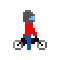###Author Topic: Tile Based Map Generation?  (Read 2084 times)

#### RKDN

• Member
•• Posts: 6
• Karma: +0/-0##### Tile Based Map Generation?
« on: Mon, Jul 30, 2012 »
I am making an RPG and I want to have randomly generated levels that are very similar to Binding of Issac.

I have no idea how to do this.

Basically I want to have a world generated at the start that is maybe 50 "rooms" wide and tall with 4 dungeons hidden somewhere in the world.

So the result that I am looking for would look similar to this (with 1's representing a room, S representing the starting location, 0's representing no room, and # representing a dungeon.

0000000000000000000#0000
000#11111110000000010000
000001000010001111110000
000001000010001001000000
000111000010001001000000
0001110000S1111111#00000
000101111110000001000000
000100000010000001110000
000#11111110000001110000
000000000000000000000000

The levels would be randomly generated so the above would only be an example.

#### finalsin

• Member
•• Posts: 51
• Karma: +0/-0##### Re: Tile Based Map Generation?
« Reply #1 on: Tue, Jul 31, 2012 »
These links might be interesting to you: http://roguebasin.roguelikedevelopment.org/index.php/Articles#Map

What you want is a bit of code that can generate an ASCII template a bit like the one you've drawn there. If you then interpret that as an array of level data passed to a FlxTileMap, most of the work is done.

1. Generate a template.
2. Convert the template into tile data so a FlxTilemap can be created.
3. Use the template to generate fixed stuff in the FlxTilemap. e.g. (pseudocode below)

Code: [Select]
`for each row in maptemplate  for each entry in row    if entry equals '#'      add new treasurechest at entry.x,y`
I dunno. Get the template generation stuff working firs,t then worry about filling the tilemap withs tuff.

#### initials##### Re: Tile Based Map Generation?
« Reply #2 on: Tue, Jul 31, 2012 »
Check out FlxCaveGenerator too.
Initials: Super Lemonade Factory, Super Lemonade Factory Part Two, Above The Clouds, Revvolvver, Four Chambers of the Human Heart#### RKDN

• Member
•• Posts: 6
• Karma: +0/-0##### Re: Tile Based Map Generation?
« Reply #3 on: Wed, Aug 1, 2012 »
I used this ( http://stackoverflow.com/questions/4997642/simple-example-of-bsp-dungeon-generation ) code as an example for the code for my random dungeon generator. The only problem I am having is using the rectangles that it creates to generate a 1 dimensional array for flixel to use as a tilemap.

it generates a series of rectangles with the properties (top, left, width, and height).

this is what I have so far... not much

Rectangle Class:
Code: [Select]
`package com { public class Rectangle { private var MIN_SIZE:uint = 5; public var top:uint; public var left:uint; public var width:uint; public var height:uint; public var leftChild:Rectangle; public var rightChild:Rectangle; private var dungeon:Rectangle; public function Rectangle(top:uint, left:uint, height:uint, width:uint) { this.top = top; this.left = left; this.width = width; this.height = height; } public function split():Boolean {        if( leftChild != null ) //if already split, bail out            return false;        var horizontal:Boolean = (Math.random() > .5) ? true : false; //direction of split        var max:int = (horizontal ? height : width ) - MIN_SIZE; //maximum height/width we can split off        if( max <= MIN_SIZE ) // area too small to split, bail out            return false;        var split:int = Math.round(Math.random()*max); // generate split point         if( split < MIN_SIZE )  // adjust split point so there's at least MIN_SIZE in both partitions            split = MIN_SIZE;        if( horizontal ) { //populate child areas            leftChild = new Rectangle( top, left, split, width );             rightChild = new Rectangle( top+split, left, height-split, width );        } else {            leftChild = new Rectangle( top, left, height, split );            rightChild = new Rectangle( top, left+split, height, width-split );        }        return true; //split successful } public function generateDungeon():void { if( leftChild != null ) { //if current are has child areas, propagate the call leftChild.generateDungeon(); rightChild.generateDungeon(); } else { // if leaf node, create a dungeon within the minimum size constraints var dungeonTop:int = (height - MIN_SIZE <= 0) ? 0 : Math.round(Math.random()*( height - MIN_SIZE)); var dungeonLeft:int =  (width - MIN_SIZE <= 0) ? 0 : Math.round(Math.random()*( width - MIN_SIZE)); var dungeonHeight:int = Math.max(Math.round(Math.random() * ( height - dungeonTop )), MIN_SIZE );; var dungeonWidth:int = Math.max(Math.round(Math.random() * ( width - dungeonLeft )), MIN_SIZE );; dungeon = new Rectangle( top + dungeonTop, left + dungeonLeft, dungeonHeight, dungeonWidth); } } }}`

The dungeonGen Class:
Code: [Select]
`package com { public class dungeonGen { public function dungeonGen() { var rectangles:Vector.<Rectangle> = new Vector.<Rectangle>(); // flat rectangle store to help pick a random one var root:Rectangle = new Rectangle( 0, 0, 60, 120 ); // rectangles.push(root); //populate rectangle store with root area while( rectangles.length < 19 ) { // this will give us 10 leaf areas var splitIdx:int = Math.round(Math.random() * ( rectangles.length - 1 )); // choose a random element var toSplit:Rectangle = rectangles[splitIdx]; if( toSplit.split() ) { //attempt to split rectangles.push( toSplit.leftChild ); rectangles.push( toSplit.rightChild ); } } root.generateDungeon(); //generate dungeons printDungeons(rectangles); } private static function printDungeons(rectangles:Vector.<Rectangle>):void { var finished:Array = new Array(); for (var i:int = 0; i < 7200; i++) { finished[i] = 0; } trace(finished); } } }`
and btw I looked around forever for some examples in as3 for Binary Space Partitioning and couldn't find any. If anyone wants to use this code feel free. I know it would have been a lot of help for me.